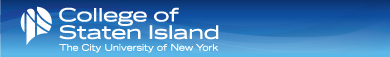VITAE PUBLICATIONS PICTURES COURSES Graph Based Analysis - GC Graph Based Analysis - CSC 769 Graph Theory - GC CSC727 CSC724 CSC513 CSC382 CSC346 CSC326 CSC228 CSC220 CSC126 CSC102COMPUTER SCIENCE DEPARTMENT

COLLEGE OF STATEN ISLAND

CITY UNIVERSITY OF NEW YORK

Louis Petingi

## Research

Network Reliability: Network components (nodes and communication links) may be subject to random failures. The reliability of a network is the probability that the network withstands these failures. A widely used probabilistic model is the one that links fail independently with known probabilities, and where the nodes are always operative. Given a network with terminal nodes K, the K-terminal Reliability of this network, is the probability that the operating links permit the terminal nodes to communicate between each other (i.e. there exists a path of operative links between every pair of nodes of K).

We've generalized this measure by restricting the paths length between every pair of K to be at most a given upper bound D. This parameter, called the Diameter-constrained K-terminal Reliability, is the probability that the network terminal nodes meet certain delay constraints.

Extremal Graph Theory: Characterize among competing topologies with equal number of nodes and edges, the ones that maximize or minimize graph theoretic indexes (e.g. number of spanning trees, connectivity, etc.)

RNA structure and prediction: RNAs secondary structures (2D) can be represented as graphs and by the application of a graph-theoretical algorithm, an RNA can be partitioned into atomic sub-structures, to detect and study for example complex structures called pseudoknots. Each of these sub-structures is stored in a library of building blocks for RNA design by fragment assembly.